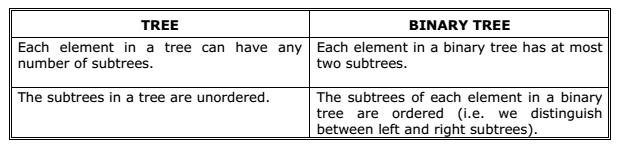# General Trees (m-ary tree) A+ A  A-

If in a tree, the outdegree of every node is less than or equal to m, the tree is called general tree. The general tree is also called as an m-ary tree. If the outdegree of every node is exactly equal to m or zero then the tree is called a full or complete m-ary tree. For m = 2, the trees are called binary and full binary trees.

Differences between trees and binary trees: